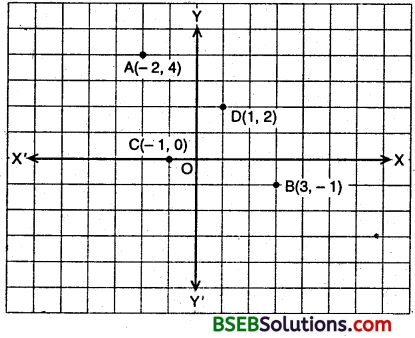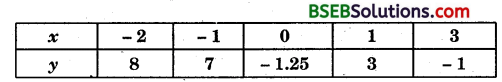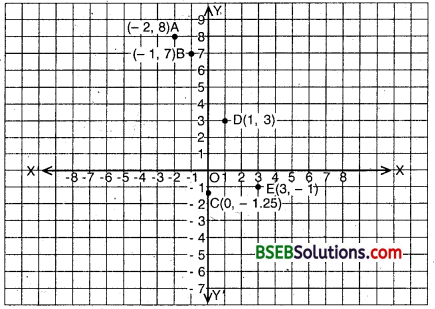# HSSlive: Plus One & Plus Two Notes & Solutions for Kerala State Board

## BSEB Class 9 Maths Chapter 3 Coordinate Geometry Ex 3.3 Textbook Solutions PDF: Download Bihar Board STD 9th Maths Chapter 3 Coordinate Geometry Ex 3.3 Book AnswersBSEB Class 9 Maths Chapter 3 Coordinate Geometry Ex 3.3 Textbook Solutions PDF: Download Bihar Board STD 9th Maths Chapter 3 Coordinate Geometry Ex 3.3 Book Answers

BSEB Class 9th Maths Chapter 3 Coordinate Geometry Ex 3.3 Textbooks Solutions and answers for students are now available in pdf format. Bihar Board Class 9th Maths Chapter 3 Coordinate Geometry Ex 3.3 Book answers and solutions are one of the most important study materials for any student. The Bihar Board Class 9th Maths Chapter 3 Coordinate Geometry Ex 3.3 books are published by the Bihar Board Publishers. These Bihar Board Class 9th Maths Chapter 3 Coordinate Geometry Ex 3.3 textbooks are prepared by a group of expert faculty members. Students can download these BSEB STD 9th Maths Chapter 3 Coordinate Geometry Ex 3.3 book solutions pdf online from this page.

## Bihar Board Class 9th Maths Chapter 3 Coordinate Geometry Ex 3.3 Books Solutions

 Board BSEB Materials Textbook Solutions/Guide Format DOC/PDF Class 9th Subject Maths Chapter 3 Coordinate Geometry Ex 3.3 Chapters All Provider Hsslive

## How to download Bihar Board Class 9th Maths Chapter 3 Coordinate Geometry Ex 3.3 Textbook Solutions Answers PDF Online?

2. Click on the Bihar Board Class 9th Maths Chapter 3 Coordinate Geometry Ex 3.3 Answers.
3. Look for your Bihar Board STD 9th Maths Chapter 3 Coordinate Geometry Ex 3.3 Textbooks PDF.
4. Now download or read the Bihar Board Class 9th Maths Chapter 3 Coordinate Geometry Ex 3.3 Textbook Solutions for PDF Free.

## BSEB Class 9th Maths Chapter 3 Coordinate Geometry Ex 3.3 Textbooks Solutions with Answer PDF Download

Find below the list of all BSEB Class 9th Maths Chapter 3 Coordinate Geometry Ex 3.3 Textbook Solutions for PDF’s for you to download and prepare for the upcoming exams:

## BSEB Bihar Board Class 9th Maths Solutions Chapter 3 Coordinate Geometry Ex 3.3

Question 1.
In which quadrant or on which axis do each of the points (- 2, 4), (3, – 1), (- 1, 0), (1, 2) and (- 3, – 5) lie? Verify your answer by locating them on the Cartesian plane.
Solution:

1. In the point (- 2, 4), abscissa is negative and ordinate is positive. So, it lies in the second quadrant.
2. In the point (3, – 1), abscissa is positive and ordinate is negative. So, it lies in the fourth quadrant.
3. The point (- 1, 0) lies on the negative x-axis.
4. In the point (1, 2) abscissa and ordinate are positive, so it lies in the first quadrant.
5. In the point (- 3, – 5) abscissa and ordinate are negative. Therefore, it lies in the fourth quadrant.

Let us locate these points on the cartesian plane. Plot the points (- 2, 4), (3, – 1), (- 1, 0) and (1, 2) as shown.These points are respectively represented by A, B, C and D, which clearly verify their location.

Question 2.
Plot the points (x, y) given in the following table on the plane choosing suitable units of distance on the axes.Solution:
Draw X’OX and Y’OY as the coordinate axes and mark their point of intersection O as the origin (0, 0).In order to plot the point (- 2, 8), we take 2 units on OX’ and then 8 units parallel to OY to obtain the point A(- 2, 8).
Similarly, we plot the point B(- 1, 7).
In order to plot (0, – 1.25), we’take 1.25 units below x-axis on they-axis to obtain C(0, – 1.25).
In order to plot (1, 3), we take 1 unit on OX and then 3 units parallel to OY to obtain the point D(l, 3).
In order to plot (3, – 1), we take 3 units on OX and then move 1 unit parallel to O’ to obtain the point E(3, – 1).

## Bihar Board Class 9th Maths Chapter 3 Coordinate Geometry Ex 3.3 Textbooks for Exam Preparations

Bihar Board Class 9th Maths Chapter 3 Coordinate Geometry Ex 3.3 Textbook Solutions can be of great help in your Bihar Board Class 9th Maths Chapter 3 Coordinate Geometry Ex 3.3 exam preparation. The BSEB STD 9th Maths Chapter 3 Coordinate Geometry Ex 3.3 Textbooks study material, used with the English medium textbooks, can help you complete the entire Class 9th Maths Chapter 3 Coordinate Geometry Ex 3.3 Books State Board syllabus with maximum efficiency.

## FAQs Regarding Bihar Board Class 9th Maths Chapter 3 Coordinate Geometry Ex 3.3 Textbook Solutions

#### Can we get a Bihar Board Book PDF for all Classes?

Yes you can get Bihar Board Text Book PDF for all classes using the links provided in the above article.

## Important Terms

Bihar Board Class 9th Maths Chapter 3 Coordinate Geometry Ex 3.3, BSEB Class 9th Maths Chapter 3 Coordinate Geometry Ex 3.3 Textbooks, Bihar Board Class 9th Maths Chapter 3 Coordinate Geometry Ex 3.3, Bihar Board Class 9th Maths Chapter 3 Coordinate Geometry Ex 3.3 Textbook solutions, BSEB Class 9th Maths Chapter 3 Coordinate Geometry Ex 3.3 Textbooks Solutions, Bihar Board STD 9th Maths Chapter 3 Coordinate Geometry Ex 3.3, BSEB STD 9th Maths Chapter 3 Coordinate Geometry Ex 3.3 Textbooks, Bihar Board STD 9th Maths Chapter 3 Coordinate Geometry Ex 3.3, Bihar Board STD 9th Maths Chapter 3 Coordinate Geometry Ex 3.3 Textbook solutions, BSEB STD 9th Maths Chapter 3 Coordinate Geometry Ex 3.3 Textbooks Solutions,
Share: